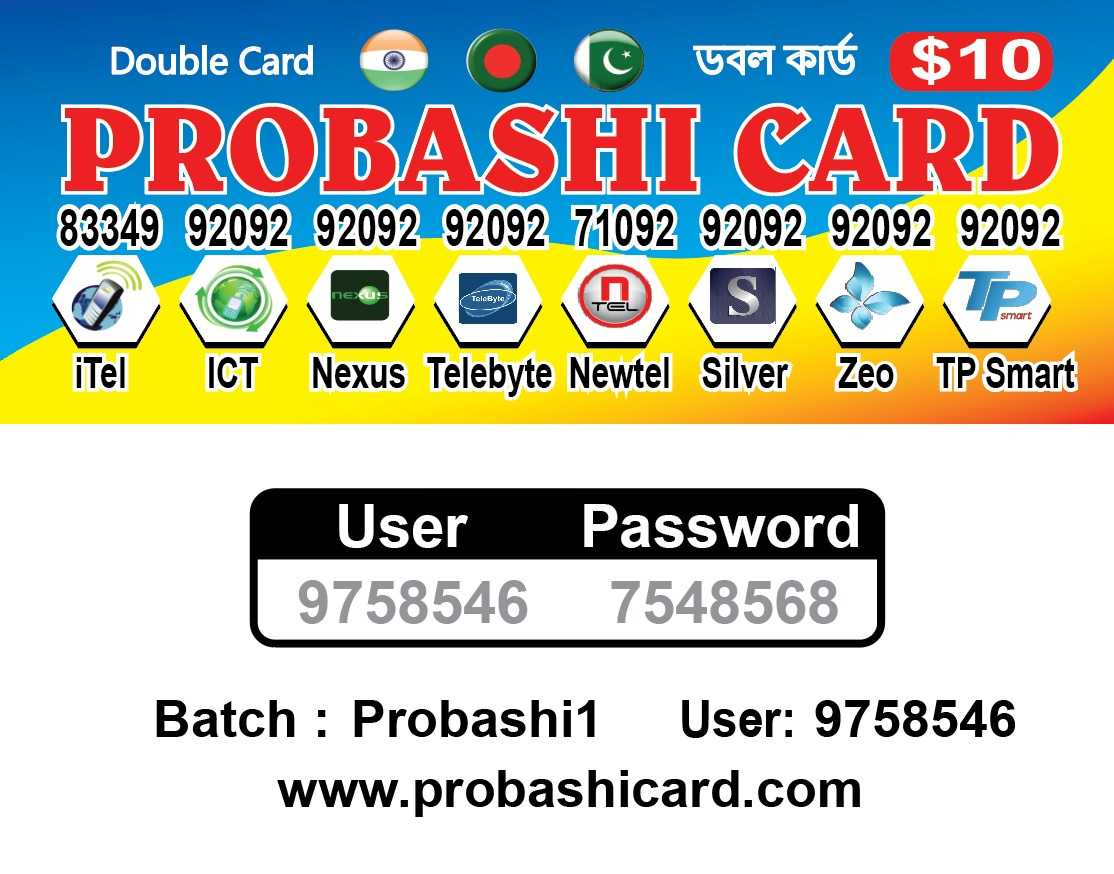3 years ago ·Translate

Rate Update-

8801=454 Min
0091=1300 min

Probashi Card

BD Gery 8801= 454 min
BD White- 008801= 200 min

India::

91= 1000 min
091= 2910 min
0091=1300 min

Pakistan Cli::

9230= 118 min
9231= 141 min
9232= 106 min
9233= 110 min
9234= 125 min

Pakistan Gery-009230=253 Min
Pakistan Gery-009231=340 Min
Pakistan Gery-009232=262 Min
Pakistan Gery-009233=279 Min
Pakistan Gery-009234= 243 min

No cuttibg....

Bdt- 350 tk(Agents)

যেকোন কোম্পানির
কলিং কার্ড প্রিন্টিং করা হয়।